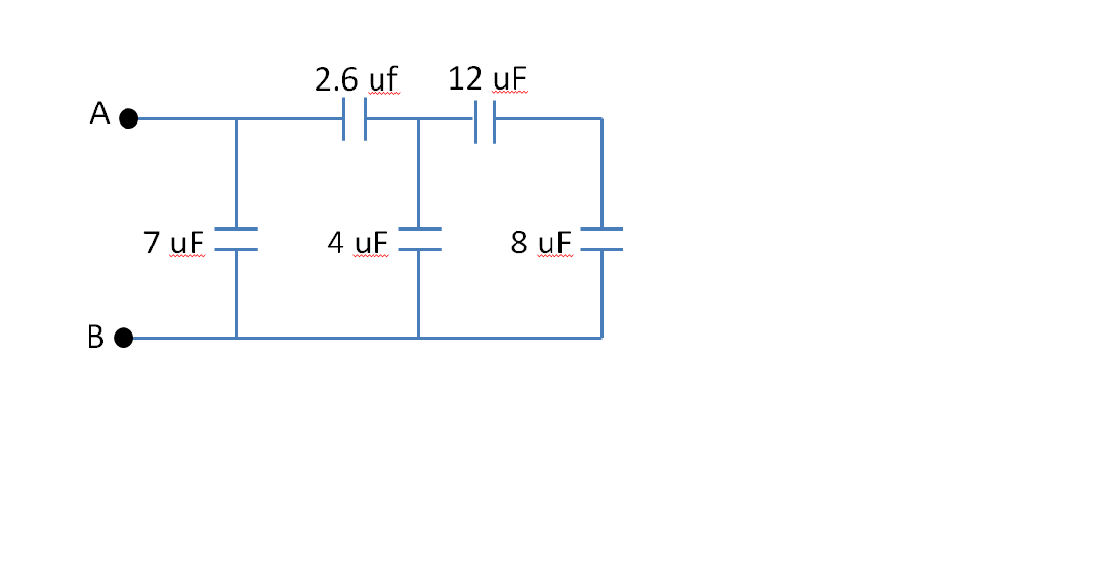Problem: Consider the circuit of capacitors shown below. The equivalent capacitance of the circuit is 9.0 μF.Determine the charge stored on the 4.0 μF capacitor. Assume that voltage across nodes A and B is 12 volts.

100% (9 ratings)
Problem Details

Consider the circuit of capacitors shown below. The equivalent capacitance of the circuit is 9.0 μF.

Determine the charge stored on the 4.0 μF capacitor. Assume that voltage across nodes A and B is 12 volts.